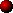STATIC GAPS

The static spark gap is the simplest type of spark gap for Tesla Coil use. Perhaps the name "Overvoltage spark gap" better describes how it operates. The term "static" arises to differentiate it from more sophisticated spark gaps which employ some moving parts. The electrodes in this type of gap are static.In its simplest form the static spark gap consists of two smooth electrodes separated by a few millimetres of air. However this often does not perform well in Tesla Coil service unless it is modified slightly. Firstly we will consider how a simple static gap works.Operation

When the voltage across the spark gap electrodes becomes sufficiently high, the air in the gap ionises, and an Avalanche effect takes place. The air inside the gap is heated to a very high temperature and becomes a good conductor of electricity. Heavy current flow through the ionised air keeps it heated and maintains the conductive channel. Eventually the current flowing will fall sufficiently that the air in the spark gap cools and stops conducting.

The avalanche process by which the spark gap starts to conduct is known as Firing or Breakdown and the voltage required to initiate this process is called the Breakdown Voltage. (The breakdown voltage is proportional to the distance between the electrodes.) During conduction the arc between the electrodes is not a perfect conductor and posses a little resistance to the flow of current through it. Power dissipated due to the arc resistance is known as conduction loss and it is the power lost here as heat that keeps the arc hot and conductive. The current necessary to keep the arc conductive is often called the holding current. When the arc cools and stops conducting it is known as quenching. The importance of quenching is explained in more detail in the section on Tesla Coil operation.

When considering how a static spark gap operates in a Tesla Coil system, the important facts are that it is "turned-on" by sufficiently high voltage, remains on due to primary current flow, and "turns-off" when the current falls too low.

Unlike a rotary spark gap, the electrodes of a static gap are always aligned. Since the static gap is voltage triggered it will fire at any time when the voltage goes above its breakdown voltage. This property is good for two reasons:

1. It prevents the primary voltage from getting too high. The static spark gap effectively limits the primary voltage by firing whenever its breakdown voltage is exceeded.
2. If the static gap is set correctly, little energy is wasted since the spark gap only fires when the tank capacitor is fully charged. Firing when the capacitor is less than fully charged would be inefficient as energy is proportional to the capacitor voltage squared.

One consequence of the constant firing voltage is that it gives rise to rather irregular timing of the spark gap firings in AC supplied systems.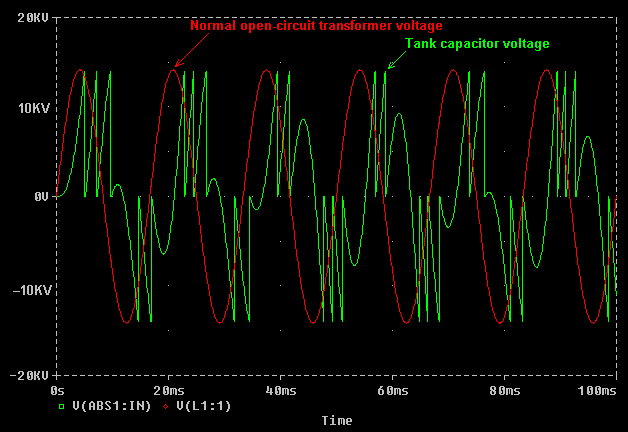In a TC supplied by AC the static gap firings tend to collect in clusters around the peaks of the supply current. This is because the tank capacitor recharges fastest when the supply current is near the peak of a cycle, and the breakdown voltage is reached in less time. In practice the firing of an AC supplied static gap system is almost always irregular, and it is quite difficult to set up the system to fire regularly over several cycles but it can be done. In terms of performance this irregular firing does not appear to hinder spark growth although it does give rise to poor power factor of the system as a whole.Static gap analysis

Effect of varying gap distance:

A useful feature of the static gap is that the total gap distance (or the number of small gaps in series,) can be adjusted to change the breakdown voltage. The three graphs below show the simulated effect of progressively making a static gap wider, in a system using a 10kv/200mA inductively ballasted supply and a "Matched" 64nF Tank capacitor.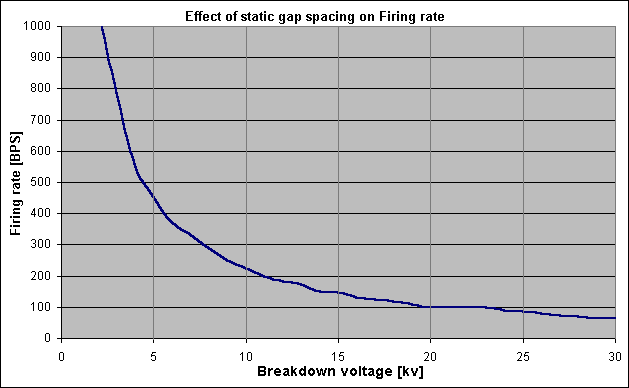The first graph shows the effect of electrode spacing on the average firing rate of the spark gap. As the static gap is made wider its breakdown voltage increases and the tank capacitor has to be charged to a higher voltage before the spark gap can fire. It takes proportionately longer for the capacitor to be charged to the higher breakdown voltages and therefore the firing rate decreases. The firing rate in Breaks per Second is approximately inversely proportional to the breakdown voltage of the spark gap. The graph above shows this relationship. (produced by Microsim PSpice simulator.)

 BPS = n / Vb Where: BPS = Firing rate in breaks per second, n = Arbitrary constant, Vb = Breakdown voltage of the spark gap.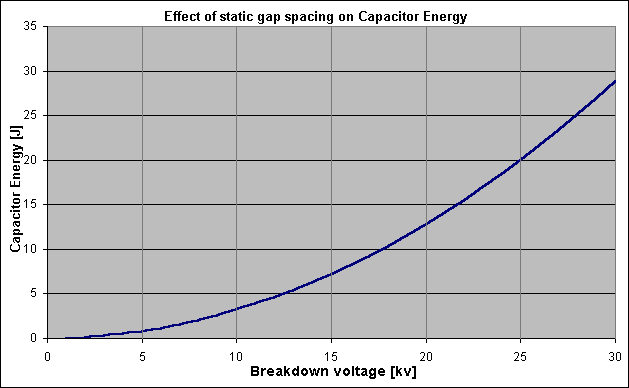The second graph shows how the energy stored in the 64nF tank capacitor increases as the spark gap is made wider. The energy stored in the tank capacitor is proportional to the breakdown voltage squared, and therefore quadruples every time the breakdown voltage of the spark gap is doubled.

 E = 0.5 x C x Vb² Where: E = Energy stored in tank capacitor (in Joules,) C = Tank capacitance is Farads, Vb = Breakdown voltage of the spark gap.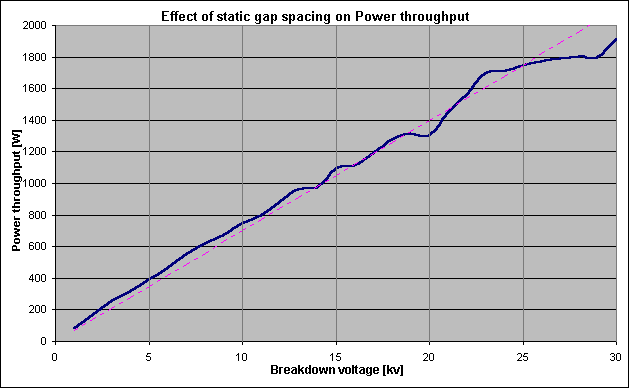Average power throughput is equal to the Firing rate multiplied by the stored capacitor energy which is released at each firing. Since the firing rate is proportional to 1/Vb and the tank energy is proportional to Vb², then it can be shown that the power throughput is roughly proportional to the breakdown voltage of the gap Vb. The simulation Graph above shows this trend. The deviations from a straight line occur due to the chaotic firing nature of the static gap.

P

= BPS x E
P
= (n / Vb) x 0.5 x C x Vb²
V
b's cancel to give:
P = 0.5 x n x C x Vb

In summary this means that both the peak voltage and the power throughput are directly proportional to the static spark gap spacing. This allows the Tesla Coil designer to control the peak voltage seen by the tank components and the average power processed simply by adjusting the total spacing of the static gap.

It is well known that wide static gaps give good performance. It is also well known that wide static gaps subject the tank capacitor and supply transformer to high voltage stresses. In the simulation above, the static gap had to be set to fire at around 30kv in order to get 2kW of power throughput with the chosen component values. This is more than twice the normal peak voltage of the 10kv supply transformer, and could easily cause dielectric failure in either the transformer or tank capacitor. The system relies on the strong resonant voltage rise effect of the charging circuit to produce sufficient voltage to breakdown the spark gap.

In practice the Static gap may be set such that its breakdown voltage is equal to the peak voltage of the supply transformer. The clamping action of the spark gap acts to protect the transformer from seeing a voltage higher than it was designed for. Unfortunately the performance is hindered slightly by setting the electrodes so close. For a given design, a compromise can usually be reached by trading-off performance against voltage stress when setting the static gap.

Effect of tank capacitor sizing:

Previous results only show the effect of varying the spark gap distance with a "Matched" capacitor of 64nF. The above simulation was repeated with a range of smaller and larger tank capacitors to find the effect of capacitor sizing on the behaviour of the system. The graph below shows how the power throughput varies with different sized tank capacitors.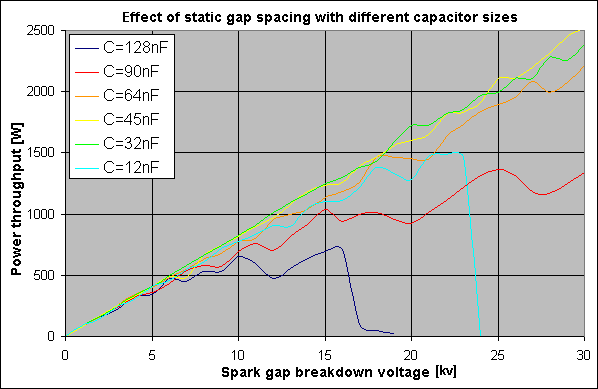Capacitors of "Matched" size or slightly smaller appear to give best performance in a static gap based system, (indicated by the Orange, Yellow and Green lines.) Capacitors which are further away from Matched size result in less resonant voltage rise in the charging circuit. For example, in the 12nF case (light blue line,) the tank capacitor voltage does not grow high enough to fire the spark gap when the gap is set to breakdown above 24kv.

In the case of the 128nF capacitor (dark blue line,) the supply is too heavily loaded by the large capacitor and is unable to charge it to a voltage of more than 17kv. If the spark gap is set to breakdown at a voltage in excess of 17kv it does not fire.

It should be realised that if the spark gap is set to fire at a voltage in excess of the peak capacitor voltage, no power will be processed into sparks, but considerable supply cord current can be drawn in order to charge the tank capacitor to the alternate peaks of the HV supply voltage. This situation can highly stress the step-up transformer if it is allowed to persist.

In summary the capacitor size does not appear to be very critical. Provided the chosen capacitor is not too small or too big, the firing rate of the system will adjust itself automatically to process the available power. Suitable capacitor values lie between 25% of Matched size and 120% of matched size. The performance is mostly dominated by the spark gap spacing alone.

Power Factor:

The above graphs all show the power throughput (or Cap watts). This is the Real Power which has made it from the supply cord through the charging circuit and into the primary tank capacitor. In practice the VA drawn from the supply cord can be significantly higher than would be expected due to the poor power factor of a static gap system.

In technical terms Power Factor is the ratio of real power to the total Volt-Amperes drawn from the supply. It can be thought of as a measure of efficiency, and describes what fraction of the supply current actually gets processed by the charging circuit and goes into making sparks. A technical description of power factor and power factor correction is given in a separate section.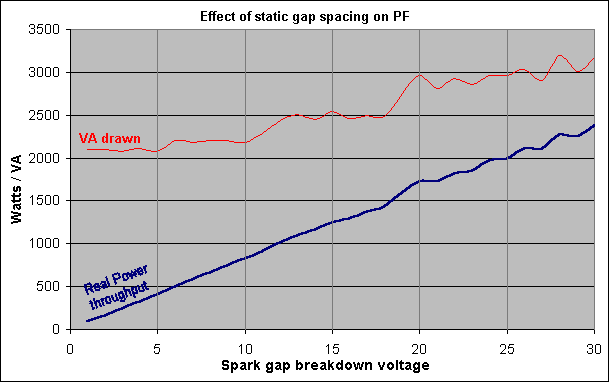The power factor of a static gap system is highly dependent on the spacing of the electrodes in the spark gap, and is only moderate at best. The graph above shows the real power and VA drawn from the supply. It can be seen that more than 2000VA is consistently drawn from the supply regardless of the gap spacing, however, a wide spacing is needed to get a significant proportion of this through the charging system as real power. In an ideal system the VA drawn would be little more than the power throughput. (For this simulation a 32nF tank capacitor was used.)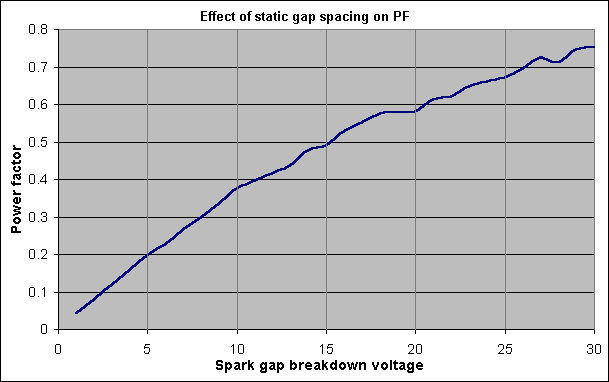Power Factor is calculated by dividing the Real Power in Watts by the total VA drawn from the supply. For small static gaps the power factor is poor and it improves steadily as the spark gap is made wider. The supply cord current can be reduced considerably by the addition of appropriate Power Factor Correction capacitors. See section on power factor.Improvements

The most basic static gap does not function very well in Tesla Coil service for several reasons, but two simple modifications can improve performance dramatically.

Modification 1,

One large air gap does not quench very well and is not often used. If several small spark gaps are connected in series then the same breakdown voltage can be achieved but the quenching ability is improved considerably. When several spark gaps are connected in series the heating is spread across more electrodes and more air gaps. This makes it easier for any one of the gaps to cool and quench. Since all gaps are connected in series, if one spark gap quenches the current flow through all of the gaps will cease. Recent investigation also suggests that connecting several spark gaps in series makes the arc unstable at low currents which may also act to improve the quenching ability of series connected gaps at primary notches.

There are, however, two slight disadvantages of dividing a spark gap into multiple smaller gaps in series. Firstly, conduction losses are higher. Although the total gap distance is comparable to one big gap, there are more arc to electrode interfaces. Since there is a fixed voltage drop at each interface the total voltage drop across the whole gap will be higher resulting in a higher power dissipation.

The second disadvantage is that the breakdown voltage of a sub-divided spark gap is less consistent than that of a single large gap. This is thought to be due to charges accumulating on the floating metal electrodes and possibly partial discharges between pairs of electrodes. This rarely poses a problem in practice, and is usually outweighed by the benefits of the multiple series gap.

A spark gap divided into several gaps is referred to as a series static gap, and often uses between 4 and 10 small gaps connected in series. Series gaps have also been made with forty or fifty very smaller gaps, but clogging of the gaps due to oxidisation can become a problem.

Modification 2,

Although the breakdown voltage is largely proportional to the electrode spacing, it reduces with increasing temperature. If the electrodes and the air in the spark gap are allowed to heat up excessively the breakdown voltage of the spark gap decreases considerably. In this instance the spark gap fires at a higher rate, but with the tank capacitor at a much reduced voltage. Since energy released from the tank capacitor is proportional to the firing voltage squared, the net power throughput drops.

This effect is responsible for the often observed gradual decrease in spark length over several seconds of Tesla Coil operation as the spark gap heats up. In severe circumstances the increased firing rate of the spark gap can cause further heating and the static gap becomes truly overloaded. A characteristic increase in pitch is noticeable in the sound from the spark gap, and it is accompanied by almost total loss of spark output.

The power handling capability of the static gap can be increased significantly by providing a high flow rate of cool clean air over the electrodes and through the air gaps. This removes hot ionised gases and also cools the electrode surfaces allowing higher power throughput before the phenomenon described above starts to occur.

Both of these modifications are used to good effect in the RQ-gap design which uses several parallel copper tube electrodes inside a cylinder. Many small air gaps are formed which are all electrically connected in series. The breakdown voltage of the RQ gap is adjusted by "tapping" at the required number of gap sections. High power fans are often mounted at the ends of the enclosure to force cooling air through the gaps and over the electrodes. Details about this very serviceable series static gap can be found on many other Tesla Coil related Web pages.Deficiencies of the static gap

In my opinion there are three major deficiencies with the static gap when considered for serious Tesla Coil work:

1. The firing time is more or less random. This makes taking repeatable measurements difficult as the behaviour is almost always different from one supply cycle to the next. Unfortunately it is difficult to make the static gap fire at a regular rate.
2. The power handling capability is limited due to the fact that the electrodes are close together and cannot effectively quench at high supply current levels.
3. When a static gap based system is first powered-up the gap is cold, and the first breakdown occurs at a higher voltage than when running. When a wide static gap is used, a very high initial voltage needs to occur before the spark gap will start firing. However, once running the firing voltage is lower and less power is being processed. As a consequence the HV transformer and primary capacitor must be rated to withstand the high power-up transient, but the performance when running is dictated by the lower breakdown voltage when running.

Fortunately most of the deficiencies of the static gap can be overcome by making the electrodes move past each other with high velocity. This is the basis of Rotary spark gaps which are covered in next few sections.Static spark gap summary

 Firing voltage: Proportional to gap spacing, Fires whenever the voltage is high enough, Firing timing: Usually very irregular, Power throughput: Directly proportional to gap spacing, Quenching: Good if lots of gaps are connected in series, Conduction losses: High if lots of gaps are placed in series, Advantages: Easy to design and build, Little set-up required, Disadvantages: Inflexible, Limited in power handling capability.

The Tesla Coil shown in the Picture Gallery on this site has a 6kV/200mA rms power supply and features a series static RQ-gap set to breakdown at 10kv. The tank capacitor is 33nF (31% of "Matched Size") and the spark gap is forced-air cooled.

Take me to the next section about Rotary spark gaps.